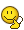# Problem with a double integral

## Homework Statement:

Compute the integral (3x dx dy), where the region of equation is : (x − 1)^2 + y^2 ≤ 1, 0 ≤ y ≤ x

## Relevant Equations:

0<=r<=2cos(θ),
I already have the solution in which the region of integration has been divided in to two regionsbut I was wondering if I can only use one region considering the polar coordinate system) the disk equation for me is r=2cos(θ) and the theta goes from 0 to (pi/4)

0<r<2cos(θ) and the 0 <θ<pi/4
the total integral becomesis my approach is correct?

Related Calculus and Beyond Homework Help News on Phys.org
Mark44
Mentor
Homework Statement:: Compute the integral (3x dx dy), where the region of equation is : (x − 1)^2 + y^2 ≤ 1, 0 ≤ y ≤ x
Relevant Equations:: 0<=r<=2cos(θ),

I already have the solution in which the region of integration has been divided in to two regions View attachment 267622
but I was wondering if I can only use one region considering the polar coordinate system) the disk equation for me is r=2cos(θ) and the theta goes from 0 to (pi/4)

0<r<2cos(θ) and the 0 <θ<pi/4
the total integral becomes
View attachment 267623

is my approach is correct?

I don't see why it wouldn't work. See if you get the same answer with both approaches. If you do, that would confirm that your approach is correct, and would also be good practice for converting integrals from Cartesian (rectangular) form to polar form.

•Amaelle
Thanks a lot for your prompt answer, the problem is that I couldn't find the same
I don't see why it wouldn't work. See if you get the same answer with both approaches. If you do, that would confirm that your approach is correct, and would also be good practice for converting integrals from Cartesian (rectangular) form to polar form.
Thanks a lot , i did it and it works!!

You can do it even without a double integral. You just need to evaluate ##\frac{1}{2}\int (2\cos{\theta})^2 d\theta##$$A = \frac{1}{2} \int_0^{\frac{\pi}{4}} 4\cos^2{\theta} \, d\theta = 2\int_0^{\frac{\pi}{4}} \frac{1}{2} + \frac{1}{2}\cos{2\theta} \, d\theta = \left[ \theta +\frac{1}{2}\sin{2\theta} \right]_0^{\frac{\pi}{4}} = \frac{\pi}{4} + \frac{1}{2}$$
thanks a lot but i think you did a miscalculation the final answer is (3pi/4)+1

•etotheipi
etotheipi
Gold Member
2019 Award
thanks a lot but i think you did a miscalculation the final answer is (3pi/4)+1
Whoops... you're right! I thought you just wanted the area